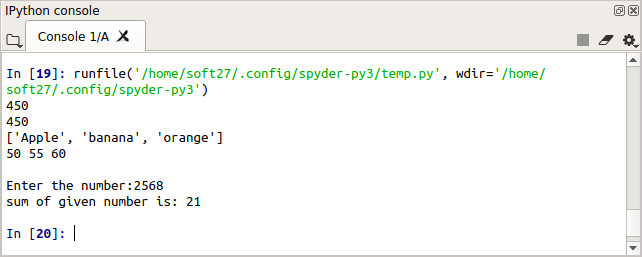• #5, First Floor, 4th Street Dr. Subbarayan Nagar Kodambakkam, Chennai-600 024 Landmark : Samiyar Madam
• pro@slogix.in
• +91- 81240 01111

### What is python statement, Indentation and comments?

###### Description

To see how type casting has been implemented in python3.

###### Multi-line statements

Python interpreter can execute are called statements.

we can make a statement extend over multiple lines with the line continuation character (\)

###### Python Indentation

Most of the programming languages like C, C++, Java use braces { } to define a block of code.

###### Python uses indentation.

The amount of indentation is up to you, but it must be consistent throughout that block.

Generally four white spaces are used for indentation and is preferred over tabs.

Comments are very important while writing a program.

It describes what's going on inside a program.

In Python, we use the hash (#) symbol to start writing a comment.

###### Sample Code

#line continuation character (\)
a = 10 + 20 + 30 + \
40 + 50 + 60 + \
70 + 80 + 90
print(a)

#explicit line continuation using (), [] and {}
b = (10 + 20 + 30 +
40 + 50 + 60 +
70 + 80 + 90)
fruits=[‘Apple’,’banana’,’orange’]
print(b)
print(fruits)

#multiple statements in single line using (;)
c=50;d=55;e=60;
print(c,d,e)

#Python indentation example(sum of digits)
#marked area is a block of code
n=int(input(“Enter the number:”))
rev=0
while(n>0):
r=n%10
rev=rev+r
n=n//10
print(“sum of given number is:”,rev)

###### Screenshots